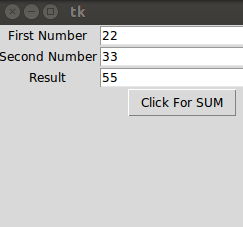# Entry TkinterEntry Tkinter is a widget to accept input from the user. Entry widget allows the user to enter information like text or numbers at the run time. In this Entry Tkinter tutorial we will learn everything about Entry to display and accept input as text from a user. Below the first program will show text box on the Tkinter window.

``````from tkinter import *
win=Tk()
win.geometry('250x250')
t1=Entry(win)
t1.grid(row=0,column=1)
win.mainloop()``````

## Entry Textbox with Label and Button

``````from tkinter import *
win=Tk()
win.geometry('250x250')

l1=Label(win,text="First Name")
l1.grid(row=0,column=0)
t1=Entry(win)
t1.grid(row=0,column=1)

l2=Label(win,text="Second Name")
l2.grid(row=1,column=0)
t2=Entry(win)
t2.grid(row=1,column=1)

b1.grid(row=2,column=1)

win.mainloop()``````

## Entry Example to Show Sum of Two Numbers

In the below example, we are using two methods get() and insert() to accept user input and print the result in Entry.

• get() – It gets the value of the Entry as text, later we can convert in any type.
• insert() – It accept two-parameter and assign value to the Entry, the first parameter is index and second is value.
``````from tkinter import *
def sum():
a=int(t1.get())
b=int(t2.get())
c=a+b
t3.insert(0,c)
win=Tk()
win.geometry('250x250')

l1=Label(win,text="First Number")
l1.grid(row=0,column=0)
t1=Entry(win)
t1.grid(row=0,column=1)

l2=Label(win,text="Second Number")
l2.grid(row=1,column=0)
t2=Entry(win)
t2.grid(row=1,column=1)

l3=Label(win,text="Result")
l3.grid(row=2,column=0)
t3=Entry(win)
t3.grid(row=2,column=1)

b1=Button(win,text="Click For SUM",command=sum)
b1.grid(row=3,column=1)

win.mainloop()``````Entry Tkinter python example to show the sum of two numbers.

In the above example, we are using get() to accept input and insert() function to show the result. We know that the entry widget accepts input as a string, so we need to typecast in the number using int() function in python.